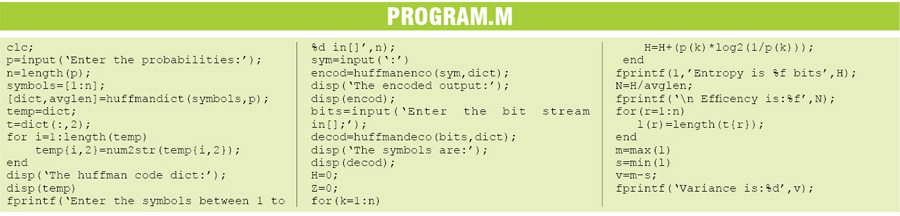Tuesday, March 21, 2023

# Huffman Encoding and Decoding in MATLAB

Nishant Mittal The author is a design engineer at Hitech Electronics, Pune. His areas of interest include MATLAB, LabVIEW, communication and embedded systems

## How MATLAB program works

1. List the source probabilities in decreasing order.

2. Combine the probabilities of the two symbols having the lowest probabilities, and record the resultant probabilities; this step is called reduction.
This procedure is repeated until there are two-order probabilities remaining.

3. Start encoding with the last reduction, which consists of exactly two-order probabilities. Assign ‘0’ as the first digit in the code words for all the source symbols associated with the first probability; assign ‘1’ to the second probability.

4. Now go back and assign ‘0’ and ‘1’ to the second digit for the two probabilities that were combined in the previous reduction step, retaining all assignments made in Step 3.

5. Keep regressing in this way until the first column is reached.

6. Calculate the entropy. The entropy of the code is the average number of bits needed to decode a given pattern.

7. Calculate efficiency. For evaluating the source code generated, you need to calculate its efficiency.

Efficiency = Entropy (H(X))/Average codeword length (N)

Average codeword length is given by:
N =∑(Pi × Ni)
where Ni is the length of ith codeword and Pi is the probability of occurence.

### MATLAB functions

Huffmanenco. This function is used in Huffman coding. The syntax is:

comp = huffmanenco(sig,dict)

This line encodes the signal ‘sig’ described by the ‘dict’ dictionary. The argument ‘sig’ can have the form of a numeric vector, numeric cell array or alphanumeric cell array. If ‘sig’ is a cell array, it must be either a row or a column. The ‘dict’ is an Nx2 cell array, where ‘N’ is the number of distinct possible symbols to be encoded. The first column of ‘dict’ represents the distinct symbols and the second column represents the corresponding codewords. Each codeword is represented as a numeric row vector, and no codeword in ‘dict’ can be the prefix of any other codeword in ‘dict’. You can generate ‘dict’ using the huffmandict function.

Huffmandeco. This function is used in Huffman decoding. The syntax is:

dsig = huffmandeco(comp,dict)

This line decodes the numeric Huffman code vector comp using the code dictionary ‘dict’. The argument ‘dict’ is an Nx2 cell array, where ‘N’ is the number of distinct possible symbols in the original signal that was encoded as ‘comp’. The first column of ‘dict’ represents the distinct symbols and the second column represents the corresponding codewords. Each codeword is represented as a numeric row vector, and no codeword in ‘dict’ is allowed to be the prefix of any other codeword in ‘dict’. You can generate ‘dict’ using the Huffmandict function and ‘comp’ using the huffmanenco function. If all signal values in ‘dict’ are numeric, ‘dsig’ is a vector; if any signal value in ‘dict’ is alphabetical, ‘dsig’ is a one-dimensional cell array.

## Testing

1. Launch the MATLAB program. The program first generates the dictionary of messages. These messages are nothing but codes or bitstreams from 00 to 1001 in this example. You can extend this range by changing in the source code. The MATLAB program output for the example is given below:

Enter the probabilities: [0.3 0.25
0.2 0.12 0.08 0.05]
The huffman code dict:
 ‘0 0’
 ‘0 1’
 ‘1 1’
 ‘1 0 1’
 ‘1 0 0 0’
 ‘1 0 0 1’
Enter the symbols between 1 to 6
in[]:
sym = 3
The encoded output:
1 1
Enter the bit stream in[];[1 1]
The symbols are: 3
Entropy is 2.360147 bits
Efficiency is 0.991659
m = 4
s = 2
Variance: 2

2. First, the program prompts you to enter the number between ‘1’ and ‘6’. When you enter ‘3’, code ‘1 1’ appears on the screen. This code is nothing but the character corresponding to number ‘3’. Hence encoding is done successfully.

3. For decoding, enter bitstream ‘1 1’. The output generated is ‘3’.

4. Instead of ‘3’, you can try out using various combinations from ‘1’ to ‘6’.The program outputs the values of maximum length (m) and minimum length (s) generated in the dictionary. The maximum length generated is ‘1111’, i.e., m=4. The minimum length is ‘00’, which is two bits long and therefore s=2.

Huffman coding is also called Minimum-variance coding. Variance is maximum length-minimum length. Hence variance is ‘2’ in this example.

Feel Interested! Check out these MATLAB Projects.

1.venkatesh

program for this huffman coding and decoding

•EFY Team

The source code is present at the end of the article.

•Arjun

The code is not working..pls help

2.Arun kohli

Plz send ne Huffman code using matlab

•EFY Team

The source code is present in the article itself.

3.THABO Gwangwa

can you please send me the Huffman code, I cant find it in the article

•EFY Team

The code is present on the second page.